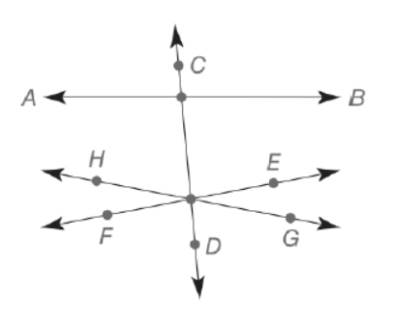Chapter 7.2, Problem 3EElementary Geometry For College St...

7th Edition
Alexander + 2 others
ISBN: 9781337614085

Solutions

Chapter
SectionElementary Geometry For College St...

7th Edition
Alexander + 2 others
ISBN: 9781337614085
Textbook Problem

Note: Exercises preceded by an asterisk are of a more challenging nature.a) In the figure, are A B ,       ↔           C D , ↔ and         E F ↔ concurrent?b) Are C D , ↔         E F ↔ , and G H ↔           concurrent?To determine

(a)

To find:

In the figure, are AB,CD, and EF concurrent.

Explanation

Definition:

Concurrent:

A number of lines are concurrent if they have exactly one point in common.

Two or more lines in a plane that have a single point in common are known as concurrent lines. When exactly two lines have a point in common, we generally describe them as intersecting lines rather than concurrent lines.

Given:

Calculation:

By the above definition,

In given figure, the three lines are not concurrent even though any pair of lines (such as ABandCD) do intersect

To determine

(b)

To find:

In the figure, are CD,EF, and GH concurrent.

Still sussing out bartleby?

Check out a sample textbook solution.

See a sample solution

The Solution to Your Study Problems

Bartleby provides explanations to thousands of textbook problems written by our experts, many with advanced degrees!

Get Started

In Exercises 2340, find the indicated limit. 26. limx23x

Applied Calculus for the Managerial, Life, and Social Sciences: A Brief Approach

Fill in each blank: 6gal3qt=pat

Elementary Technical Mathematics

In Exercises 1-6, simplify the expression. 818

Calculus: An Applied Approach (MindTap Course List)

Rational Inequalities Solve the inequality. 30. 1x3+1x+22xx2+x2

Precalculus: Mathematics for Calculus (Standalone Book)

Which curve is simple but not closed?

Study Guide for Stewart's Multivariable Calculus, 8th

True or False: ln(a + b) = ln a + ln b.

Study Guide for Stewart's Single Variable Calculus: Early Transcendentals, 8th